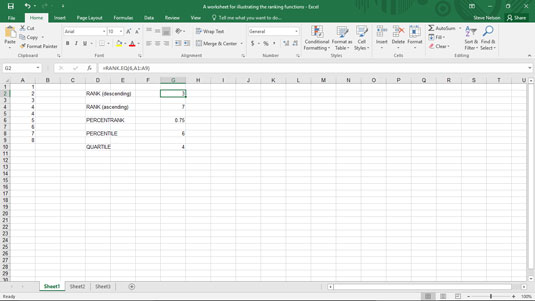##### Excel Data Analysis For DummiesThe PERCENTRANK.EXC and PERCENTRANK.INC functions in Excel determine the percentage rank, or percentile, of a value in an array. You use the PERCENTRANK.EXC function to determine the percentage rank exclusive of the first and last values in the array, and you use the PERCENTRANK.INC function to determine the percentage rank inclusive of the first and last values in the array. Both formulas use the same arguments.

The PERCENTRANK.EXC formula uses the syntax

`=PERCENTRANK.EXC(<i>array</i>,<i>x</i>,[<i>significance</i>])`

The PERCENTRANK.INC formula uses the syntax

`=PERCENTRANK.INC(<i>array</i>,<i>x</i>,[<i>significance</i>])`

where array gives the array of values, x identifies the value you want to rank, and significance identifies the number of decimal places that you want in the percentage. The significance argument is optional. If you omit the argument, Excel assumes that you want three significant digits.

To demonstrate how the PERCENTRANK.INC function works, again suppose you want to rank the values shown in the worksheet range A1:A9 in the figure — only this time, you rank the values using percentages.A worksheet fragment with the array 1, 2, 3, 4, 4, 5, 6, 7, 8.

The formula in cell G6

`=PERCENTRANK.INC(A1:A9,6,2)`

returns the value 0.75, which is the same thing as 75 percent.

Excel calculates the percentage rank by looking at the number of array values greater than the x value and the number of array values smaller than the x value. The array shown in the figure includes the values 1, 2, 3, 4, 4, 5, 6, 7, 8. The percent rank of 6 in the array equals 0.75 because six array values are smaller than 6 and two array values are larger than 6. The actual formula that the function calculates is 6/(2+6), which equals 0.75.